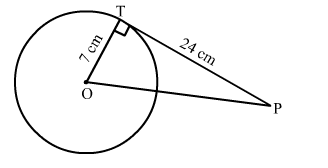# In a circle of radius 7 cm, tangent PT is drawn from a point P, such that PT = 24 cm.`
Question:

In a circle of radius 7 cm, tangent PT is drawn from a point P, such that PT = 24 cm. If O is the centre of the circle, then OP = ?(a) 30 cm
(b) 28 cm
(c) 25 cm
(d) 18 cm

Solution:

(c) 25 cm
The tangent at any point of a circle is perpendicular to the radius at the point of contact.

$\therefore O T \perp P T$

From right $-$ angled triangle $P T O$,

$\therefore O P^{2}=O T^{2}+P T^{2}[$ Using Pythagoras' theorem $]$

$\Rightarrow O P^{2}=(7)^{2}+(24)^{2}$

$\Rightarrow O P^{2}=49+576$

$\Rightarrow O P^{2}=625$

$\Rightarrow O P=\sqrt{625}$

$\Rightarrow O P=25 \mathrm{~cm}$